# System Pumping Requirements

Another simple way to do this is to estimate the maximum crop water use rate (in/day) you need to keep up with, and convert this to gpm/acre (in/day x 18.9 = gpm/acre). (Precipitation rate conversions here). You then need to divide by the irrigation application efficiency as a decimal (such as 0.75). Many irrigation system designers in Eastern Washington use 6.0 to 7.5 gpm/acre. Go here for a calculator for your crop, soil, and area. This gpm/acre is then just multiplied by the field size to get a system design flow rate in gpm.

Net Irrigation Depth Required Per Week:
Total Area:
Total Hours Available to Operate per Day:
hrs
Total Days Available to Operate per Week:
days per week
Irrigation Efficiency:
%
Total Flow Required to Operate System:

## The Equation

This calculator uses this formula to determine the system pumping requirements of a field.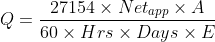Where: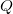= Total flow rate required to operate irrigation system (gpm)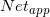= The net application required per week (in)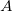= Total area to be irrigated (acres)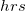= Total hours available to operate per day (hrs)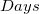= Total days available to operate each week (days)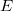= Efficiency of the irrigation system (as a deciaml)

WSU Prosser - IAREC, 24106 N Bunn Rd, Prosser WA 99350-8694, 509-786-2226. Contact Us